# KSEEB Solutions for Class 6 Maths Chapter 5 Understanding Elementary Shapes Ex 5.8

Students can Download Chapter 5 Understanding Elementary Shapes Ex 5.8 Questions and Answers, Notes Pdf, KSEEB Solutions for Class 6 Maths helps you to revise the complete Karnataka State Board Syllabus and score more marks in your examinations.

## Karnataka State Syllabus Class 6 Maths Chapter 5 Understanding Elementary Shapes Ex 5.8

Question 1.
Examine whether the following are polygons. If any one among them is not, say why?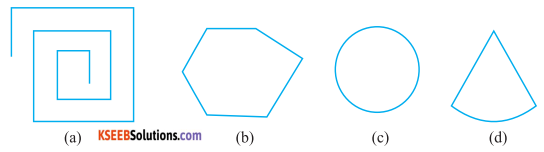Solution:
a) It is not a polygon as it is not a closed figure
b) Yes, it is a polygon made of 6 sides
c) No, it is not made of line segments.
d) No, it is not made of only line segmentsQuestion 2.
Name each polygon.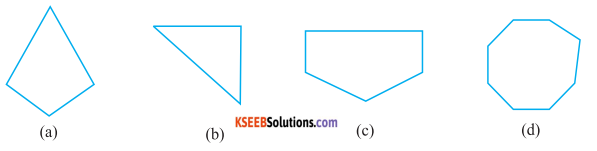Make two more examples of each of these.
Solution:
a) The given figure is a Quadrilateral as this closed figure is made of 4
Line segments two more example are.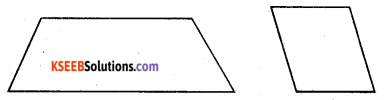b) The given figure is a triangle as this closed figure is made of 3 line segments two more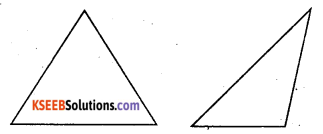c) The given figure is a pentagon as this closed figure is made of 5 line segments two more example are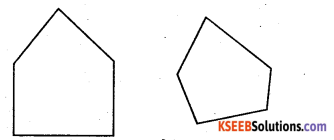d) The give figure is an octagon as this closed figure is made of 8 fine segments Two more examples are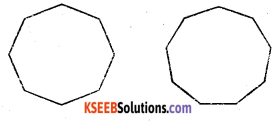Question 3.
Draw a rough sketch of a regular hexagon. Connecting any three of its vertices, draw a triangles identify the type of the triangle you have drawn.
Solution:
An isosceles triangle by joining three of the vertices of a hexagon can be drawn as follows.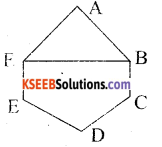ABCDEF is a required octagon.

Question 4.
Draw a rough sketch of a regular octagon, (Use squared paper if you wish) Draw a rectangle by joining exactly four of the vertices of the octagon.
Solution: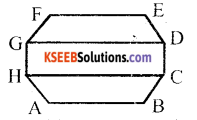ABCDEFGH is a required octagon.Question 5.
A diagonal is a line segment that joins any two vertices of the polygon and is not a side of the polygon. Draw a rough sketch of a pentagon and draw its diagonals.
Solution: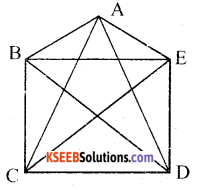It can be observed here that AC, AD, BD, BE, CE are the diagonals